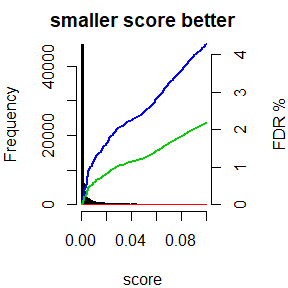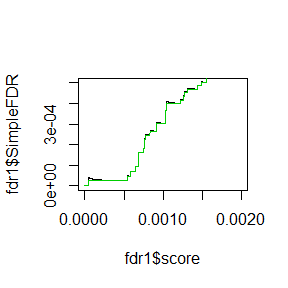# Target Decoy FDR

## The data

The package provides sample data. The data needs to have two columns, one with a label, to distinguish targets and decoys, the other with a score. Then you also need to know if a larger score or a smaller score is better. In our example data, we have two scores: score and score2. While the score is better if it smaller, score2 is better if it is larger.

rm(list=ls())
library(dplyr)
library(prozor)
data(fdrSample)
x <-dplyr::arrange(fdrSample, score2)
knitr::kable(head(fdrSample))
proteinID score score2
sp|P0C0L4|CO4A_HUMAN 0.0000008 6.121995
sp|P0C0L4|CO4A_HUMAN 0.0000023 5.640072
sp|P0C0L4|CO4A_HUMAN 0.0288739 1.539495
sp|P0C0L4|CO4A_HUMAN 0.0019398 2.712251
sp|P0C0L4|CO4A_HUMAN 0.0000001 7.231987
sp|P0C0L4|CO4A_HUMAN 0.0000063 5.203215

What is also required is that the number of Targets and Decoys are the same. This is usually given for mass spectrometry database searches. Our dataset here is truncated already at a 5% FDR. Therefore the number of decoys is much smaller.

table(grepl("REV_",fdrSample$proteinID)) ## ## FALSE TRUE ## 88782 1933 In our example, we will use the package to filter the data further for a 1% FDR. Computing the FDR can be done by calling the function computeFDR. plotFDR than shows the score distribution for the targets (black) and decoys (red) as well as the FDR for each score (x axis). fdr1 <- computeFDR(fdrSample$score, grepl("REV_",fdrSampleproteinID),larger_better = FALSE) plotFDR(fdr1)The output is a named list which can be easily converted into a data frame. We next will briefly discuss the elements of the output. knitr::kable(head(data.frame(fdr1))) larger_better order decoy_hit score FPR SimpleFDR qValue_FPR qValue_SimpleFDR FALSE 26160 FALSE 0 0 0 0 0 FALSE 26265 FALSE 0 0 0 0 0 FALSE 26156 FALSE 0 0 0 0 0 FALSE 26158 FALSE 0 0 0 0 0 FALSE 26253 FALSE 0 0 0 0 0 FALSE 26286 FALSE 0 0 0 0 0 ## The order column Since the scores are sorted to compute the FDR, we return also the order column. This column can be used to align the ID’s with the scores. knitr::kable(head(data.frame(ID = fdrSampleproteinID[fdr1$order], fdr1))) ID larger_better order decoy_hit score FPR SimpleFDR qValue_FPR qValue_SimpleFDR sp|P04264|K2C1_HUMAN FALSE 26160 FALSE 0 0 0 0 0 sp|P35527|K1C9_HUMAN FALSE 26265 FALSE 0 0 0 0 0 sp|P04264|K2C1_HUMAN FALSE 26156 FALSE 0 0 0 0 0 sp|P04264|K2C1_HUMAN FALSE 26158 FALSE 0 0 0 0 0 sp|P35527|K1C9_HUMAN FALSE 26253 FALSE 0 0 0 0 0 sp|P35527|K1C9_HUMAN FALSE 26286 FALSE 0 0 0 0 0 For convinience we provide the function computeFDRwithID which integrates the reordering of the ID’s. fdr1 <- computeFDRwithID(fdrSample$score,fdrSample$proteinID, decoy = "REV_",larger_better = FALSE) knitr::kable(head(data.frame(fdr1))) larger_better order decoy_hit score FPR SimpleFDR qValue_FPR qValue_SimpleFDR ID FALSE 26160 FALSE 0 0 0 0 0 sp|P04264|K2C1_HUMAN FALSE 26265 FALSE 0 0 0 0 0 sp|P35527|K1C9_HUMAN FALSE 26156 FALSE 0 0 0 0 0 sp|P04264|K2C1_HUMAN FALSE 26158 FALSE 0 0 0 0 0 sp|P04264|K2C1_HUMAN FALSE 26253 FALSE 0 0 0 0 0 sp|P35527|K1C9_HUMAN FALSE 26286 FALSE 0 0 0 0 0 sp|P35527|K1C9_HUMAN ## Types of FDR There are various types of measures in result dataframe. We define here  * FP as the number of passing decoy assignments * TP as the number of passing forward hits. ### False postive rate Which is the “Fraction of incorrect assignments above score threshold”. The multiplier 2 is needed here since we assume that also the forward sequences have false assignments. $FPR = \frac{2 \cdot FP}{TP + FP}$ This is taken from the reference by (Elias and Gygi 2007). (Storey and Tibshirani 2003) defines FPR differently. Kaell points out that the FPR here actually should be named FDR and that “Many proteomics papers incorrectly refer to this quantity as the false positive rate.” . ### Simple FDR “The FDR associated with a particular score threshold is defined as the expected percentage of accepted PSMs that are incorrect, where an accepted PSM is one that scores above the threshold (Many proteomics papers incorrectly refer to this quantity as the false positive rate).” ((Käll et al. 2007)) The Simple FDR intrudced by ((Käll et al. 2007)) is defined by : $SimpleFDR = \frac{FP}{TP}$ “For a given score threshold, we count the number of decoy PSMs above the threshold and the number of target PSMs above the threshold. We can now estimate the FDR by simply computing the ratio of these two values (SimpleFDR).”(Käll et al. 2007) plot(fdr1$score, fdr1$SimpleFDR, type="l", xlim=c(0,0.002), ylim=c(0,0.0005)) lines(fdr1$score, fdr1\$qValue_SimpleFDR, col=3, type="l", xlim=c(0,0.002), ylim=c(-0.002,0))However, although the score is getting better (smaller) the FDR may increase since the number of TP in the denominator decreases while the number of FP stays the same. Therefore Storey and Tibshirani proposed the q-value, “which in our case is defined as the minimal FDR threshold at which a given PSM is accepted”(Käll et al. 2007).

## Getting the score for an FDR.

Most frequently you will need to get the score for an FDR in order to filter your data. To report your data with an FDR of 1% instead of 5% you can execute this code:

names(fdr1)
##  "larger_better"    "order"            "decoy_hit"
##  "score"            "FPR"              "SimpleFDR"
##  "qValue_FPR"       "qValue_SimpleFDR" "ID"
(score01G <- predictScoreFDR(fdr1,qValue = 5,method = "FPR"))
##  0.09996855
dim(dplyr::filter(fdrSample, score < score01G))
##  90714     3
(score01G <- predictScoreFDR(fdr1,qValue = 1,method = "FPR"))
##  0.007166919
dim(dplyr::filter(fdrSample, score < score01G))
##  64469     3
(score01K <- predictScoreFDR(fdr1,qValue = 1,method = "SimpleFDR"))
##  0.02797633
dim(dplyr::filter(fdrSample, score < score01K))
##  77266     3